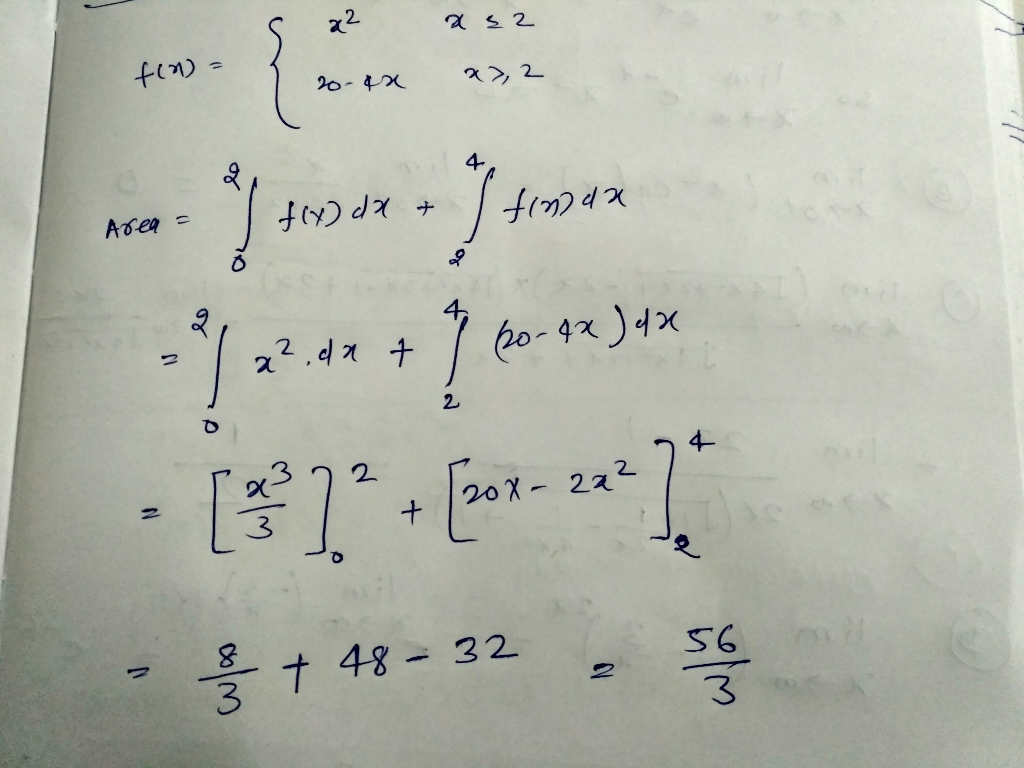# Find the area under the graph of f over the interval [0,4]. f(x) = x^2 for x< or equal to 2 20...

Find the area under the graph of f over the interval [0,4].

f(x) = x^2 for x< or equal to 2

20-4x for x>2##### Add Answer of: Find the area under the graph of f over the interval [0,4]. f(x) = x^2 for x< or equal to 2 20...
More Homework Help Questions Additional questions in this topic.

• #### 2^2x-1 = 32^x-2 2/x - 1/2x= 1/2 (2 over x minus 1 over 2x equals 1 over 2) 3/x + 8/x-5= 1 (3 over x plus 8 over x minus 5 equals 1)

Need Online Homework Help?